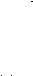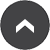Year 4 - Mr Dawes

Spring Term Maths Overview

07 Jan 2016Spring 1st Half Term. Maths Overview.

 Wk Weekly Summary Strands Objectives 11 Place 4-digit numbers on landmarked lines; 0–10 000 and 1000–2000; round 4-digit numbers to the nearest 10, 100 and 1000; mentally add and subtract to/from 4-digit and 3-digit numbers using place-value; count on and back in multiples of 10, 100 and 1000; count on in multiples of 25 and 50; add and subtract multiples of 10 and 100 to/from 4-digit numbers Number and place value (NPV) NPV.45 Understand place value in 4-digit numbers by creating 4-digit numbers, placing them on a number line and solving place value additions and subtractions NPV.49 Round 4-digit numbers up or down to the nearest 10, 100 or 1000 NPV.52 Use place value to add and subtract multiples of 10, 100 and 1000 to and from 4-digit numbers NPV.41 Count on and back in 50s NPV.43 Count above 1000 in 1s and 100s NPV.44 Count beyond 1000 in 10s NPV.50 Count in 1s, 10s and 100s, across multiples of 100 and 1000 NPV.51 Count in 50s and 25s, using the 100s count Problem solving, reasoning and algebra (PRA) PRA.52 Describe, predict and explain patterns 12 Use expanded written subtraction and compact written subtraction to subtract pairs of 3-digit numbers (one ʻexchangeʼ); use expanded column subtraction and compact column subtraction to subtract pairs of 3-digit and 2-digit numbers from 3-digit numbers (one ʻcarryʼ); learn the 7× table and ʻtrickyʼ facts; use the vertical algorithm to multiply 3-digit numbers by 1-digit numbers; solve simple money problems with decimals to two decimal places Written addition and subtraction (WAS) WAS.48 Use expanded decomposition to subtract 3-digit from 3-digit numbers WAS.50 Use compact decomposition to subtract 3-digit from 3-digit numbers WAS.51 Subtract 2-digit from 3-digit numbers using expanded or compact decomposition Mental multiplication and division (MMD) MMD.31 Understand that multiplication is commutative and use it in mental calculations MMD.52 Count in 7s and recall multiplication and division facts for the ×7 table Written multiplication and division (WMD) WMD.49 Multiply 2- and 3-digit by 1-digit numbers using the ladder method Problem solving, reasoning and algebra (PRA) PRA.58 Solve simple measure and money problems involving fractions and decimals up to 2 decimal places Measurement (MEA) MEA.61 Estimate, compare and calculate different measures, including money in pounds and pence 13 Use mental multiplication and division strategies; find non-unit fractions of 2-digit and 3-digit numbers; find equivalent fractions and use them to simplify fractions (halves, thirds, quarters) Mental multiplication and division (MMD) MMD.41 Use doubling and halving to multiply and divide by 4 and 8 and solve correspondence problems MMD.49 Double and halve 3-digit numbers by partitioning MMD.55 Use mental strategies to solve multiplications including multiplying by 0 and 1, dividing by 1, multiplying together three numbers MMD.57 Use mental strategies to solve divisions including dividing by 1 Fractions, ratio and proportion (FRP) FRP.38 Find fractions of amounts and relate to division and multiplication FRP.41 Understand unit and non-unit fractions with denominators ≤ 12 FRP.46 Develop an understanding of equivalence in fractions; 1/2s, 1/3s, 1/4s, 1/5s, 1/6s, 1/8s, 1/10s FRP.52 Identify the equivalent fraction for any given fraction FRP.34 Begin to understand equivalence by placing fractions on a number line FRP.54 Use equivalence to reduce fractions to their simplest form Problem solving, reasoning and algebra (PRA) PRA.58 Solve simple measure and money problems involving fractions and decimals up to 2 decimal places 14 Recognise and compare acute, right and obtuse angles; draw lines of a given length; identify perpendicular and parallel lines; recognise and draw line symmetry in shapes; sort 2D shapes according to their properties; draw shapes with given properties and explain reasoning; draw the other half of symmetrical shapes Geometry: properties of shapes (GPS) GPS.54 Estimate and measure angles, recognising that they are measured in degrees GPS.56 Compare and classify acute and obtuse angles; order angles up to 180° GPS.43 Draw horizontal, perpendicular and parallel lines of a given length GPS.44 Identify line symmetry in 2D shapes presented in different orientations GPS.49 Classify 2D shapes according to their properties: right angles, lines of symmetry, parallel and perpendicular lines GPS.51 Draw shapes with specified properties: a right angle, two perpendicular lines, two parallel lines GPS.47 Recognise and begin to complete symmetrical 2D shapes GPS.60 Complete a symmetric figure with a given line of symmetry Problem solving, reasoning and algebra (PRA) PRA.53 Use, explain and justify mathematical reasoning 15 Understand how to divide 2-digit and 3-digit numbers by 1-digit numbers using place value and mental strategies; divide numbers by 1-digit numbers to give answers between 10 and 25, with remainders; identify factor pairs and use these to solve multiplications and divisions with larger numbers; use Frog to find complements to multiples of 1000; use Frog to find change from £10, £20 and £50 Mental multiplication and division (MMD) MMD.37 Understand division as the inverse of multiplication MMD.61 Identify factors and multiples, and begin to find common factors MMD.64 Use knowledge of multiples and factors in relation to large numbers Written multiplication and division (WMD) WMD.51 Divide 2- and 3-digit by 1-digit numbers using a written method drawn from mental strategies with integer remainders and answers between 10 and 20 Mental addition and subtraction (MAS) MAS.50 Subtract 4-digit from 4-digit multiples of 1000 by counting up MAS.57 Use number facts to add to the next multiple of 100 or 1000 MAS.40 Find change from £5, £10 and £20 by counting up MAS.51 Count up to find change from £10, £50 and £100 Problem solving, reasoning and algebra (PRA) PRA.52 Describe, predict and explain patterns

Go to the page-top